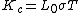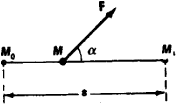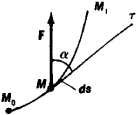# work

(redirected from work over)
Also found in: Dictionary, Thesaurus, Medical, Financial, Idioms.

## work

work, in physics and mechanics, transfer of energy by a force acting to displace a body. Work is equal to the product of the force and the distance through which it produces movement. Although both force and displacement are vector quantities, having both magnitude and direction, work is a scalar quantity, having only magnitude. If the force acts in a direction other than that of the motion of the body, then only that component of the force in the direction of the motion produces work. Thus when a 5-lb (22.4-newton) force pulls a body 10 ft (3 m), it does 50 foot-pounds (67.2 meter-newtons) of work. If a force acts on a body constrained to remain stationary, no work is done by the force. Even if the body is in motion, the force must have a component in the direction of motion. Thus, any centripetal force, such as the sun's gravitational pull on the earth, does no work because it acts at right angles to the motion and has no component in that direction (see centripetal force and centrifugal force). When there is no friction and a force acts on a body, the work done by the force is equal to the increase of the kinetic and potential energy of the body, since all the energy expended by the agency exerting the force must be gained by the body. If frictional forces are present, then some of the work must go to overcome friction and appears finally in the form of heat energy. A simple machine is a device for converting work into another form of energy. For example the jackscrew converts an input of work done on the machine to raise the load. The efficiency of a machine, which is defined as the ratio of the work output to the work input, is always less than one, since some of the input is invariably wasted in overcoming friction. The element of time does not enter into the computation of work; the time rate of doing work is called power. One horsepower is an expenditure of 33,000 foot-pounds per minute. Some of the units used to measure work are the foot-pound, the erg, and the joule.

## Work

In physics, the term work refers to the transference of energy that occurs when a force is applied to a body that is moving in such a way that the force has a component in the direction of the body's motion. Thus work is done on a weight that is being lifted, or on a spring that is being stretched or compressed, or on a gas that is undergoing compression in a cylinder.

When the force acting on a moving body is constant in magnitude and direction, the amount of work done is defined as the product of just two factors: the component of the force in the direction of motion, and the distance moved by the point of application of the force. Thus the defining equationfor work W is given below, where f and s are the magnitudes of the force and displacement, respectively, and &phgr; is the angle between these two vector quantities (see illustration). Because f cos &phgr; · s = f · s cos &phgr; work may be defined alternatively as the product of the force and the component of the displacement in the direction of the force.

The work done is positive in sign whenever the force or any component of it is in the same direction as the displacement; one then says that work is being done by the agent exerting the force and on the moving body. The work is said to be negative whenever the direction of the force or force component is opposite to that of the displacement; then work is said to be done on the agent and by the moving body. From the point of view of energy, an agent doing positive work is losing energy to the body on which the work is done, and one doing negative work is gaining energy from that body.

The work principle, which is a generalization from experiments on many types of machines, asserts that, during any given time, the work of the forces applied to the machine is equal to the work of the forces resisting the motion of the machine, whether these resisting forces arise from gravity, friction, molecular interactions, or inertia.

The work done by any conservative force, such as a gravitational, elastic, or electrostatic force, during a displacement of a body from one point to another has the important property of being path-independent: Its value depends only on the initial and final positions of the body, not upon the path traversed between these two positions. On the other hand, the work done by any nonconservative force, such as friction due to air, depends on the path followed and not alone on the initial and final positions, for the direction of such a force varies with the path, being at every point of the path tangential to it. See Energy, Force

McGraw-Hill Concise Encyclopedia of Physics. © 2002 by The McGraw-Hill Companies, Inc.

## work

see SOCIOLOGY OF WORK.
Collins Dictionary of Sociology, 3rd ed. © HarperCollins Publishers 2000
The following article is from The Great Soviet Encyclopedia (1979). It might be outdated or ideologically biased.

## Work

(1) The work of a force is a measure of the action of the force. It depends on the magnitude and direction of the force and on the displacement of the force’s point of application. If a force F is constant in magnitude and direction and the displacement M0M1 is rectilinear (Figure 1), then the work A = F ⋅s ⋅ cos α, where s = M0 M1 and α is the angle between the direction of the force and that of the displacement. When α ≤ 90°, the work is positive; when 180° ≥ α > 90°, it is negative; and when o = 90°, that is, the force is perpendicular to the displacement, A = 0. The units of measurement of work are the joule (J), the erg (1 erg = 10–7 J), and the kilogram-meter (1 kilogram-meter = 9.81 J).Figure 1

In general, the calculation of work involves the concept of incremental work dA = Fdscos α, where ds is an infinitesimal displacement and α is the angle between the direction of the force and the tangent τ to the displacement path at the point of the force’s application (Figure 2). In Cartesian coordinates,

(1) dA = Fxdx + Fydy + Fzdz

where Fx, Fy, and Fz are the projections of the force on the coordinate axes, and x, y, and ζ are the coordinates of the point of application of the force. In generalized coordinates,

(2) dA = Ʃi δqi

where the qi are generalized coordinates and the Qi are generalized forces. For forces acting on a body having a fixed axis of rotation, dA = Mzdɸ, where Mz is the sum of the moments of the forces about the axis of rotation and ɸ is the angular displacement. For pressure forces, dA = pdV, where ρ is the pressure and V is the volume.Figure 2

The work of a force through a finite displacement is defined as the integral of the incremental elements of work. For a displacement M0M1, the work is expressed by the curve integral

A = ʃ M0M1 (F cos α) ds

or

A = ʃ M0M1 (Fx dx + Fy dy + Fz dz)

For work performed by gradient vector fields, d π— d π l and A = Π0 – Π1 where Π0 and Π1 are the values of the potential energy Π at the initial and final positions of the system. In this case, the work is independent of the displacement path. With the motion of a mechanical system, the sum of the work of all forces acting through a displacement is equal to the change in the system’s kinetic energy T; that is,

ΣAi - T1- T0

The concept of work is used extensively in mechanics and in other branches of physics and engineering.

S. M. TARG

(2) Work in thermodynamics is a generalization of the concept of work in mechanics as expressed in differential form in equation (2). The generalized coordinates in thermodynamics are the external parameters of a thermodynamic system. Examples are the system’s position in space and volume and the intensity of an external magnetic or electric field. Here, the generalized forces, such as pressure, are quantities that depend not only on the coordinates but also on the internal parameters of the system (temperature or entropy). The work performed by a thermodynamic system on external bodies consists in the change of state of these bodies and is determined by the amount of energy imparted to external bodies by the system upon a change in the system’s external parameters. In equilibrium adi-abatic processes, the work is equal to the change in the system’s internal energy; in equilibrium isothermal processes, it is equal to the change in free energy (Helmholtz free energy). In many cases, the work can be expressed in terms of other thermodynamic potentials.

In general, the amount of work performed in the transition of a system from an initial state to a final state depends on the path through which the transition is accomplished. This means that an infinitesimal increment of work by the system is not a total differential of some function of the system’s state. An incremental amount of work is therefore usually designated not by the total differential dA but by δA. Since the amount of work depends on the path, it is possible that the work will not be equal to zero when a system completes a cycle. This discrepancy is important to the operation of all heat engines.

The work of external forces on a system is δA’ = - δ A if the energy of the system’s interaction with the external bodies does not change during the performance of the work. Examples of work arising from a change in one of the external parameters of a system are seen in the work performed by the forces of external pressure ρ in changing the volume V of a system, where SA = pdV, in the work of the forces of surface tension in changing a system’s surface, where δA = – σdΣ, where σ is the surface tension coefficient and dΣ is a surface element, and in the work of the magnetization of a system δA = – HdJ, where H is the intensity of the external magnetic field and J is the magnetic moment per unit volume of a body. The work of a system in a nonequilibrium (irreversible) process is always less than in an equilibrium process. From a statistical viewpoint, work in thermodynamics is the change in the average energy of a system as a result of a change in the system’s energy levels, while the change in energy in heat transfer is connected with a change in the probability of filling the energy levels.

### REFERENCES

Leontovich, M. A. Vvedenie ν termodinamiku, 2nd ed. Moscow-Leningrad, 1952.
Reif, F. Statisticheskaia fizika. (Berkleevskii kurs fiziki, vol. 5.) Moscow, 1972. (Translated from English.)

G. IA. MIAKISHEV

The Great Soviet Encyclopedia, 3rd Edition (1970-1979). © 2010 The Gale Group, Inc. All rights reserved.

## What does it mean when you dream about work?

As an activity that occupies a large part of our lives, a dream about work can simply be a reflection of our everyday experiences. Perhaps a work dream can be symbolizing other kinds of efforts, such as “working” on one’s marriage. The term “work” is also a part of several dozen different idioms, from “dirty work” and a “nasty piece of work” to making “short work” of something and “working one’s fingers to the bone.”

## work

[wərk]
(electricity)
(industrial engineering)
The physical or mental effort expended in the performance of a task.
(mechanics)
The transference of energy that occurs when a force is applied to a body that is moving in such a way that the force has a component in the direction of the body's motion; it is equal to the line integral of the force over the path taken by the body.
McGraw-Hill Dictionary of Scientific & Technical Terms, 6E, Copyright © 2003 by The McGraw-Hill Companies, Inc.

## work

1. All labor necessary to produce the construction required by the contract documents, and all materials and equipment incorporated or to be incorporated in such construction.
2. The produce of a force by its corresponding displacement.
McGraw-Hill Dictionary of Architecture and Construction. Copyright © 2003 by McGraw-Hill Companies, Inc.

## work

1. something done, made, etc., as a result of effort or exertion
2. any piece of material that is undergoing a manufacturing operation or process; workpiece
3. decoration or ornamentation, esp of a specified kind
4. an engineering structure such as a bridge, building, etc.
5. Physics the transfer of energy expressed as the product of a force and the distance through which its point of application moves in the direction of the force.
Collins Discovery Encyclopedia, 1st edition © HarperCollins Publishers 2005

## work

That which is the result of labor. A work may be written text of any kind, a graphic image, an audio recording, a video clip, a motion picture, choreography, sculpture, a painting, music, architecture or program source code.
Copyright © 1981-2019 by The Computer Language Company Inc. All Rights reserved. THIS DEFINITION IS FOR PERSONAL USE ONLY. All other reproduction is strictly prohibited without permission from the publisher.
Site: Follow: Share:
Open / Close